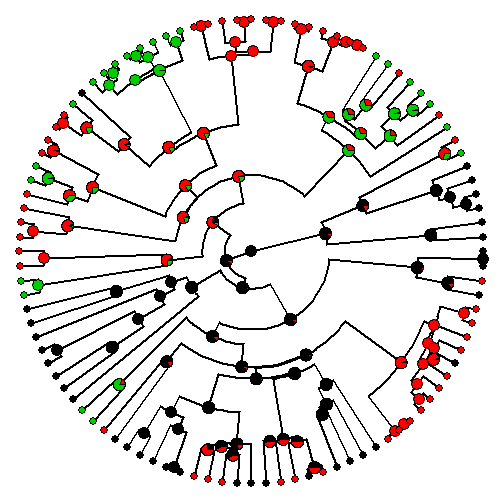## Thursday, September 17, 2015

### Important updates to internal function used by make.simmap

I just rewrote the function that is used internally to compute the likelihood of any transition matrix `Q` given the data & tree; and to estimate the ML value of `Q`. This function is highly similar (but slightly different in important ways), both functionally & structurally, to `ace(...,type="discrete")` in the ape package. It is also in some ways functionally redundant with `ace` and `fitDiscrete` in that they fit the same model - although they differ in that the prior distribution on the root node can be controlled in this function. In fact, this is the main reason for this 're-write' because in previous versions of phytools, I suspect that this prior was being used improperly in the calculations. Now, when this function is used internally by `make.simmap` (which is it's main purpose, although it can also be used alone) the prior, `pi`, can influence not only the states sampled at the root (and thus, indirectly, for other nodes in the tree), but it can also affect the estimated value of `Q` under likelihood.

Here's a quick demo of using the function alone to fit the Mk discrete character evolution model:

``````library(devtools)
install_github("liamrevell/phytools",quiet=TRUE)
``````

Simulate tree & data:

``````library(phytools)
tree<-pbtree(n=100,scale=1)
Q<-matrix(c(-1,1,0,1,-2,1,0,1,-1),3,3)
rownames(Q)<-colnames(Q)<-letters[1:3]
Q
``````
``````##    a  b  c
## a -1  1  0
## b  1 -2  1
## c  0  1 -1
``````
``````x<-sim.history(tree,Q,anc="a")\$states
``````
``````## Done simulation(s).
``````
``````x
``````
``````##  t16  t17  t63  t64  t39  t29  t54  t55   t5  t18  t50  t51  t33  t19  t20
##  "a"  "a"  "a"  "a"  "a"  "a"  "c"  "b"  "c"  "b"  "c"  "c"  "c"  "b"  "c"
##  t10  t99 t100  t88  t76  t79  t80  t93  t94  t89  t90  t44  t95  t96  t68
##  "c"  "b"  "b"  "b"  "b"  "b"  "b"  "b"  "b"  "b"  "b"  "b"  "b"  "b"  "c"
##  t69  t65  t81  t82  t77  t78  t60   t9  t40  t83  t84  t72  t73  t56  t57
##  "c"  "c"  "c"  "c"  "c"  "c"  "c"  "a"  "c"  "b"  "b"  "b"  "b"  "c"  "c"
##  t26  t21  t61  t62  t42  t43  t52  t53   t6   t7   t2  t58  t59  t11  t12
##  "b"  "b"  "b"  "b"  "b"  "b"  "c"  "c"  "a"  "a"  "a"  "a"  "a"  "a"  "a"
##   t1  t22  t23   t3   t4  t45  t46  t27  t85  t86  t35  t36  t37  t38  t28
##  "a"  "c"  "c"  "b"  "a"  "a"  "a"  "a"  "a"  "a"  "b"  "b"  "b"  "a"  "a"
##  t24  t25  t30  t74  t75  t14  t15  t13   t8  t87  t91  t92  t70  t71  t49
##  "a"  "b"  "b"  "a"  "b"  "a"  "a"  "a"  "a"  "b"  "b"  "b"  "b"  "b"  "b"
##  t47  t48  t41  t34  t66  t67  t31  t32  t97  t98
##  "b"  "a"  "b"  "b"  "b"  "b"  "a"  "b"  "a"  "a"
``````

Fit the model:

``````obj<-fitMk(tree,as.factor(x),model="SYM")
obj
``````
``````## Object of class "fitMk".
##
## Fitted (or set) value of Q:
##           a         b        c
## a -0.930216  0.930216  0.00000
## b  0.930216 -2.094586  1.16437
## c  0.000000  1.164370 -1.16437
##
## Fitted (or set) value of pi:
##         a         b         c
## 0.3333333 0.3333333 0.3333333
##
## Log-likelihood: -66.577856
``````

It is also used internally by `make.simmap`. Here, with a prior that is not flat:

``````trees<-make.simmap(tree,x,pi=setNames(c(1,0,0),letters[1:3]),nsim=100)
``````
``````## make.simmap is sampling character histories conditioned on the transition matrix
## Q =
``````
``````##            a          b        c
## a -0.8965016  0.8965016  0.00000
## b  0.8965016 -2.0898515  1.19335
## c  0.0000000  1.1933498 -1.19335
``````
``````## (estimated using likelihood);
## and (mean) root node prior probabilities
## pi =
``````
``````## a b c
## 1 0 0
``````
``````## Done.
``````
``````obj<-summary(trees)
obj
``````
``````## 100 trees with a mapped discrete character with states:
##  a, b, c
##
## trees have 34.17 changes between states on average
##
## changes are of the following types:
##      a,b a,c  b,a  b,c c,a  c,b
## x->y  12   0 6.36 9.63   0 6.18
##
## mean total time spent in each state is:
##               a         b         c    total
## raw  12.1857664 8.5412422 4.3792713 25.10628
## prop  0.4853673 0.3402034 0.1744293  1.00000
``````
``````plot(obj,ftype="off",type="fan")
``````That's it.